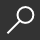## Maryland 6th Grade Math Test Prep : Common Core Learning Standards by Teachers' Treasures

Overview -

Our 1st edition Maryland 6th Grade Math Test Prep for Common Core State Standards is an excellent resource to assess and manage student's understanding of concepts outlined in the Common Core State Standards Initiative. This resource is formatted into three sections: Diagnostic, Practice, and Assessment with multiple choice in each section. The material covered includes understanding ratio concepts and using ratio reasoning to solve problems; applying and extending previous understandings of multiplication and division to divide fractions by fractions; computing fluently with multi-digit numbers and find common factors and multiples; applying and extending previous understandings of numbers to the system of rational numbers; applying and extending previous understandings of arithmetic to algebraic expressions; reasoning about and solving one-variable equations and inequalities; representing and analyzing quantitative relationships between dependent and independent variables; solving real-world and mathematical problems involving area, surface area, and volume; and developing an understanding of statistical variability. These standards are covered extensively by the practice problems. This book contains over 1000 practice problems aligned to each Common Core State Standard. In addition the book contains an answer key to practice problems.
Paperback: 358 double-sided pages
Publisher: Teachers' Treasures, Inc.
Language: English

local_shippingFor DeliveryIn Stock.
This item is Non-Returnable.
FREE Shipping for Club Members helpNew & Used Marketplace 3 copies from \$22.56

## More About Maryland 6th Grade Math Test Prep by Teachers' Treasures

### Overview

Our 1st edition Maryland 6th Grade Math Test Prep for Common Core State Standards is an excellent resource to assess and manage student's understanding of concepts outlined in the Common Core State Standards Initiative. This resource is formatted into three sections: Diagnostic, Practice, and Assessment with multiple choice in each section. The material covered includes understanding ratio concepts and using ratio reasoning to solve problems; applying and extending previous understandings of multiplication and division to divide fractions by fractions; computing fluently with multi-digit numbers and find common factors and multiples; applying and extending previous understandings of numbers to the system of rational numbers; applying and extending previous understandings of arithmetic to algebraic expressions; reasoning about and solving one-variable equations and inequalities; representing and analyzing quantitative relationships between dependent and independent variables; solving real-world and mathematical problems involving area, surface area, and volume; and developing an understanding of statistical variability. These standards are covered extensively by the practice problems. This book contains over 1000 practice problems aligned to each Common Core State Standard. In addition the book contains an answer key to practice problems.
Paperback: 358 double-sided pages
Publisher: Teachers' Treasures, Inc.
Language: English

This item is Non-Returnable.

### Details

• ISBN-13: 9781505715491
• ISBN-10: 1505715490
• Publisher: Createspace Independent Publishing Platform
• Publish Date: December 2014
• Page Count: 360
• Dimensions: 11 x 8.5 x 0.75 inches
• Shipping Weight: 1.84 poundsRelated Categories